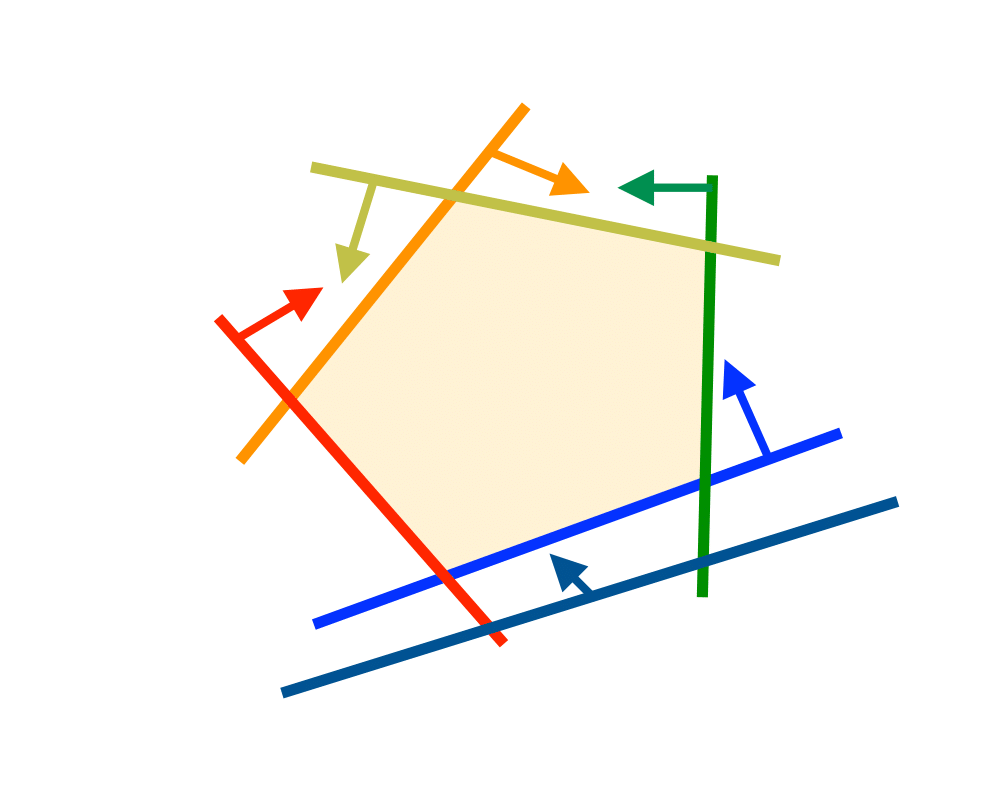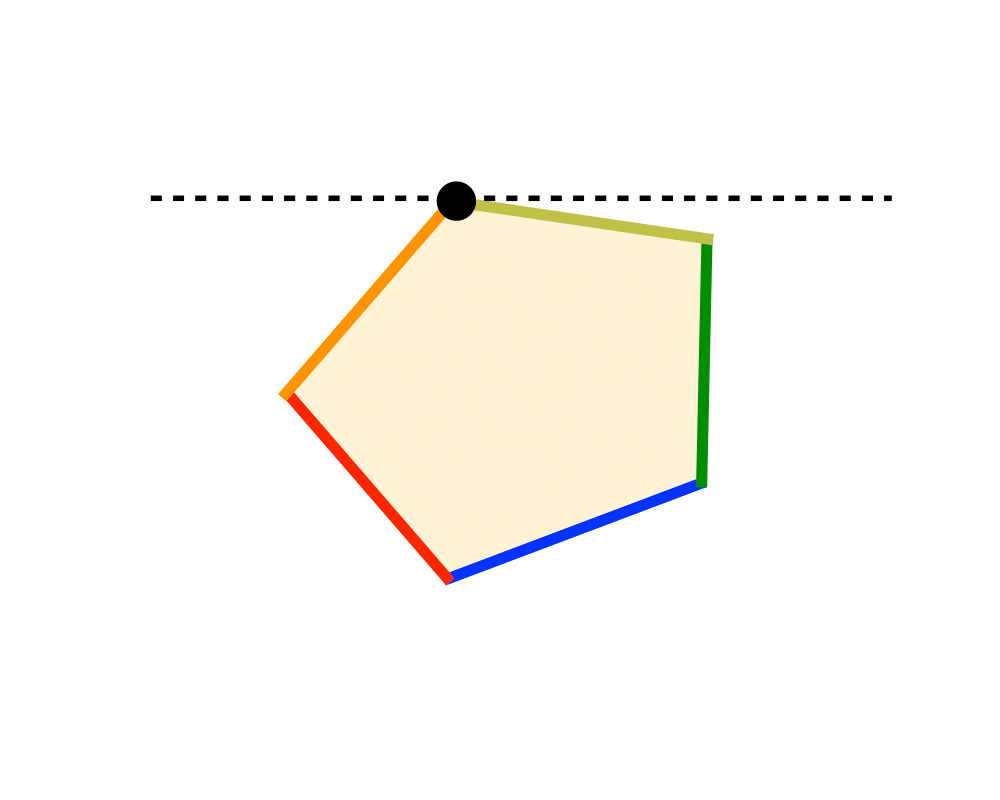# Linear ProgrammingInputOutput

Input Description: A set of linear inequalities, a linear objective function.
Problem: Find the assignment to the variables maximizing the objective function while satisfying all inequalities.

Excerpt from The Algorithm Design Manual: The standard algorithm for linear programming is called the simplex method. Each constraint in a linear programming problem acts like a knife that carves away a region from the space of possible solutions. We seek the point within the remaining region that maximizes (or minimizes) $$f(X)$$. By appropriately rotating the solution space, the optimal point can always be made to be the highest point in the region. Since the region (simplex) formed by the intersection of a set of linear constraints is convex, we can find the highest point by starting from any vertex of the region and walking to a higher neighboring vertex. When there is no higher neighbor, we are at the highest point.

While the basic simplex algorithm is not too difficult to program, there is a considerable art to producing an efficient implementation capable of solving large linear programs. For example, large programs tend to be sparse (meaning that most inequalities use few variables), so sophisticated data structures must be used. There are issues of numerical stability and robustness, as well as which neighbor we should walk to next (so called pivoting rules). Finally, there exist sophisticated interior-point methods, which cut through the interior of the simplex instead of walking along the outside, that beat simplex in many applications.

Go To Main Page